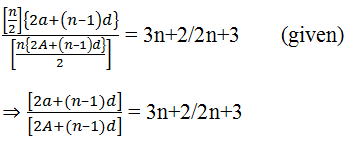×#### Thank you for registering.

One of our academic counsellors will contact you within 1 working day.

Click to Chat

1800-1023-196

+91-120-4616500

CART 0

• 0

MY CART (5)

Use Coupon: CART20 and get 20% off on all online Study Material

ITEM
DETAILS
MRP
DISCOUNT
FINAL PRICE
Total Price: Rs.

There are no items in this cart.
Continue Shopping```Sum of first n terms of APA sequence of numbers <an> is said to be in arithmetic progression if the difference between any two terms of the sequence is constant. The constant difference between the two terms is called the common difference.

If ‘a’ is the first term of the sequence and ‘d’ is the common difference then the nth term of A.P. is given by

tn = a + (n-1)d

In this case, the sum of first n terms of the A.P. is given by:

Sn = n/2 [2a + (n-1)d]

Watch this Video for more reference

We discuss some of the problems based on arithmetic progression:

Illustration: Let sum of n terms of a series be n (2n–1). Find its mth term.

Solution: Let Sm and Sm–1 denote the sum of first m and (m – 1) terms respectively.

Sm = T1 + T2 + T3 + ……. + Tm–1 + Tm

Sm–1 = T1 + T2 + T3 + ……. + Tm–1

Subtracting the two equations

Sm – Sm–1 = Tm

⇒ Tm = (m(2m–1))–(m–1)(2(m–1)–1))

= (2m2 – m)–(2m2 – 5m + 3)

= 4m – 3

Illustration: The sum of n terms of two A.P.’s is in the ratio 3n+2: 2n+3. Find the ratio of their 10th terms.

Solution: let us assume the two A.P’s to be of the forms

Let a, a + d, a + 2d, a + 3d, ……………

A, A + D, A + 2D, A + 3D, ………………It is given in the question that

⇒ To get the ratio of 10th terms put n–1/2 = 9

or n = 19

⇒ a+9d/A+9D = 3(19)+2/2(19)+3 = 59/41

Illustration: Let Tr be the rth term of an A.P. for r =1, 2, 3, ….. if for some positive integers m and n we have Tm = 1/n and Tn = 1/m, then find the value of Tmn.

Solution: Let Tm = a + (m-1)d = 1/n

Tn = a + (n-1)d = 1/m

On subtracting the second equation from the first equation, we get,

(m-n)d = 1/n – 1/m =(m-n)/mn

Hence, this gives d = 1/mn

Again, Tmn = a + (mn-1)d

= a+(mn – n + n -1)d

= a+(n-1)d+(mn-n)d

= Tn +n(m-1)1/mn

= 1/m + (m-1)/m =

Hence Tmn = 1.

Illustration: The fourth power of the common difference of an A.P. with integer entries is added to the product of any four consecutive terms of it. Prove that the resulting sum is the square of an integer.

Solution: Let four consecutive terms of the A.P. a-3d, a-d, a+d, a+3d

Then the product is given by

P = (a-3d)(a-d)(a+d)(a+3d) + (2d)4

= (a2-9d2) (a2-d2) + 16d4

= (a2-5d2)2

Now, (a2-5d2) = a2-9d2 + 4d2

(a-3d)(a+3d) + (2d)2

= I.I + I2

= I

Therefore, P = I2 = Integer.

askIITians extends to students the opportunity of getting answers from IIT experts on any kind of queries relating to arithmetic progressions or finding the sum of n terms of A.P.

Related Resources

Look into the Sample Papers with Solutions to get a hint of the kinds of questions asked in the exam.

To read more, Buy study materials of Sequences and Series comprising study notes, revision notes, video lectures, previous year solved questions etc. Also browse for more study materials on Mathematics here.
```### Course Features

• 731 Video Lectures
• Revision Notes
• Previous Year Papers
• Mind Map
• Study Planner
• NCERT Solutions
• Discussion Forum
• Test paper with Video Solution